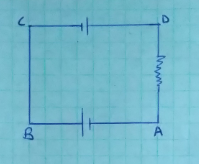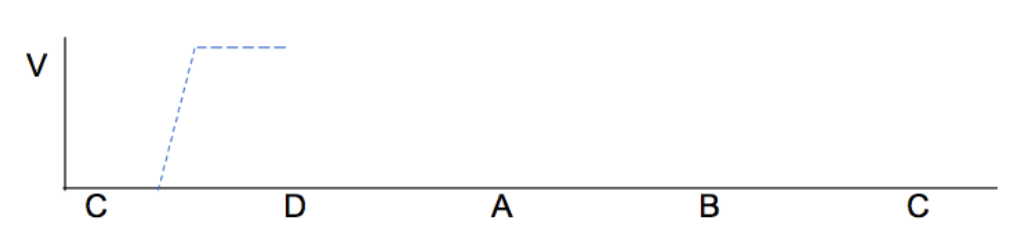# Problem: 2. (1 pt) Sketch, in a plot like the one below, the voltage as one moves around the outer loop in the circuit from part A, from point C through V1 to point D, through R1 to A, through V2 to B, then back to C. Assume point C is at zero volts. Label specific voltages on the y-axis; in other words, the graph should show quantitatively the change in voltages through this loop. The graph has been started to you (dashed line).

###### FREE Expert Solution

Let's first show the circuit as follows:85% (347 ratings)###### Problem Details

2. (1 pt) Sketch, in a plot like the one below, the voltage as one moves around the outer loop in the circuit from part A, from point C through V1 to point D, through R1 to A, through V2 to B, then back to C. Assume point C is at zero volts. Label specific voltages on the y-axis; in other words, the graph should show quantitatively the change in voltages through this loop. The graph has been started to you (dashed line).